# 论文笔记：Bottom-Up and Top-Down Attention for Image Captioning and Visual Question Answering

## Bottom-Up Attention Model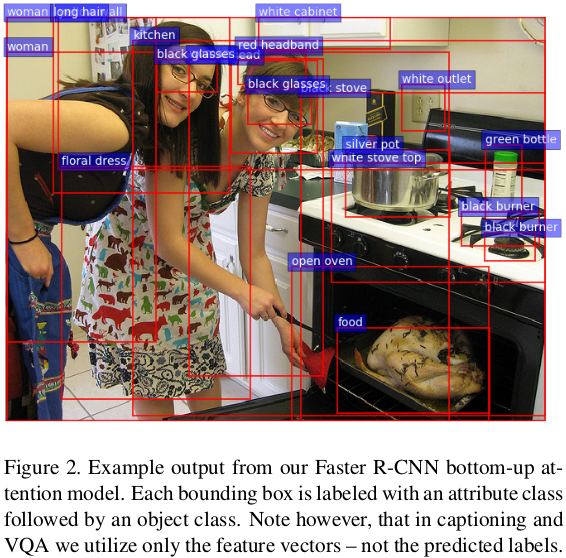bottom up attetion model在完成imageNet的预训练后，又到visual genome数据上进行了训练，原因如下。

## Image Caption部分

Caption model结构如图所示，共有2个LSTM模块，一个是Language LSTM，另一个是Top-Down Attention LSTM。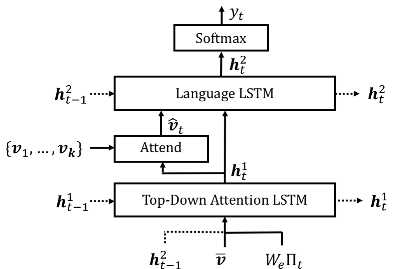${h}_{t}=LSTM\left({x}_{t},{h}_{t-1}\right)$$h_t = LSTM(x_t, h_{t-1})$

### top-down attention LSTM

top-down attention使用LSTM来确定image feature ${v}_{i}$$v_i$ 的权重, 是soft attention机制。

1. 前一时刻language model的hidden state ${h}_{t-1}^{2}$$h^2_{t-1}$
2. k个image feature的 mean-pooled feature $\overline{v}=\frac{1}{k}\sum _{i}{v}_{i}$$\overline{v} = \frac{1}{k}\sum_iv_i$
3. 之前生成的词的encoding（${\Pi }_{t}$$Π_t$为t时刻输入单词的one-hot encoding，${W}_{e}$$W_e$为embedding矩阵。)

PS:论文原文提到:
These inputs provide the attention LSTM with maximum context regarding the state of the language LSTM, the overall content of the image, and the partial caption output generated so far, respectively.

${a}_{i,t}={w}_{a}^{T}tanh\left({W}_{va}{v}_{i}+{W}_{ha}{h}_{t}^{1}\right)$$a_{i,t} = w_a^T tanh(W_{va} v_i + W_{ha} h_t^1)$

${\alpha }_{t}=softmax\left({a}_{t}\right)$$\alpha_t = softmax(a_t)$

$\stackrel{^}{{v}_{t}}=\sum _{i=1}^{K}{\alpha }_{i,t}{v}_{i}$$\hat{v_t} = \sum_{i=1}^K\alpha_{i,t}v_i$

### language LSTM

language LSTM的输入${x}_{t}^{2}=\left[\stackrel{^}{{v}_{t}},{h}_{t}^{1}\right]$$x_t^2 = [ \hat{v_t}, h^1_t]$由上述的：

1. attention feature加权和$\stackrel{^}{{v}_{t}}$$\hat{v_t}$
2. 当前时刻attention LSTM的hidden state ${h}_{t}^{1}$$h^1_t$
concatenate而成。

t 时刻输出的任一单词的概率分布$p\left({y}_{t}|{y}_{1:t-1}\right)=softmax\left({W}_{p}{h}_{t}^{2}+{b}_{p}\right)$$p(y_t|y_{1:t-1})=softmax(W_p h^2_t +b_p)$，其中$\left({y}_{1},...,{y}_{T}\right)$$(y_1,...,y_T)$为单词的序列。

### 训练目标

${L}_{R}\left(\theta \right)=-{\mathbb{E}}_{{y}_{1:T}\sim {p}_{\theta }}\left[r\left({y}_{1:T}\right)\right]$$L_R(\theta) = -\mathbb{E}_{y_{1:T}\sim p_\theta} [r(y_{1:T})]$

${\mathrm{\nabla }}_{\theta }{L}_{R}\left(\theta \right)\approx \left(r\left({y}_{1:T}^{S}\right)-r\left({\stackrel{^}{y}}_{1:T}\right)\right){\mathrm{\nabla }}_{\theta }\mathrm{log}{p}_{\theta }\left({y}_{1:T}^{S}\right)$$\nabla_\theta L_R(\theta) \approx (r(y^S_{1:T}) - r(\hat y_{1:T}))\nabla_\theta \log p_\theta(y^S_{1:T})$

## VQA部分

VQA模型也同样使用的是 soft top-down attention机制，结构如图所示：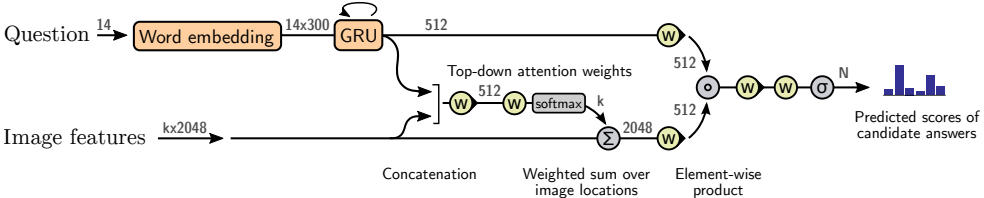$\stackrel{~}{y}=tanh\left(Wx+b\right)$$\tilde y = tanh (Wx + b)$

$g=\sigma \left({W}^{\prime }x+b\right)$$g = \sigma (W'x+b)$

$y=\stackrel{~}{y}\circ g$$y = \tilde y \circ g$

VQA中的问题Q被编码成GRU内的隐状态$q$$q$，其中单词是以可训练的word embedding来表达的。

${a}_{i}={w}_{a}^{T}{f}_{a}\left(\left[{v}_{i},q\right]\right)$$a_i = w^T_a f_a([v_i,q])$

$h={f}_{q}\left(q\right)\circ {f}_{v}\left(\stackrel{^}{v}\right)$$h = f_q(q)\circ f_v(\hat v)$

$p\left(y\right)=\sigma \left({W}_{o}{f}_{o}\left(h\right)\right)$$p(y) = \sigma(W_of_o(h))$

### 实验结果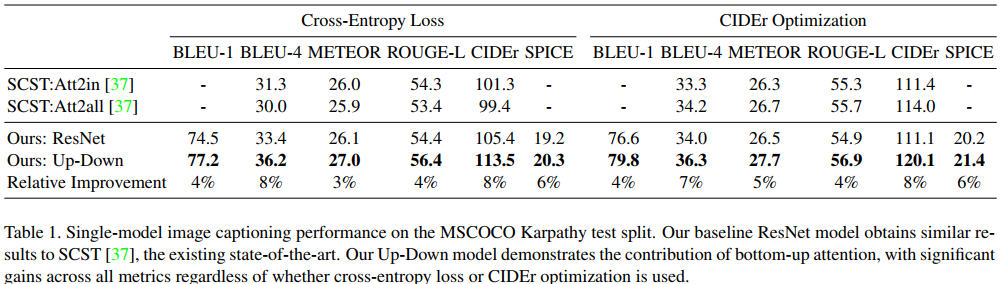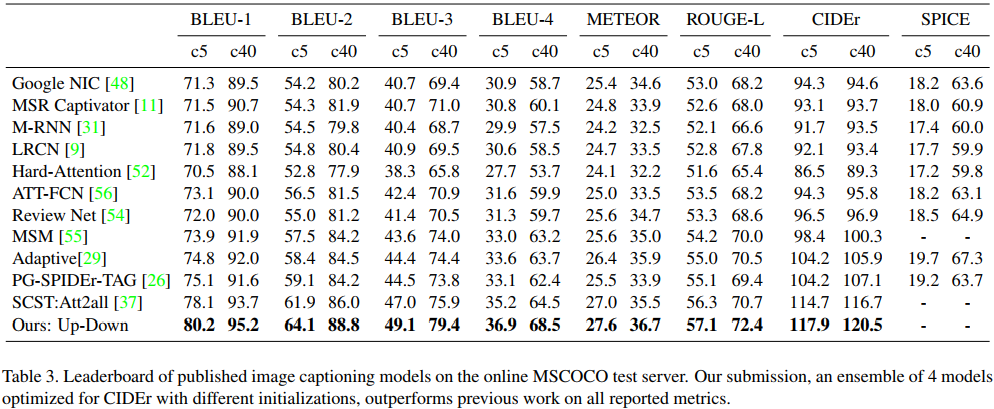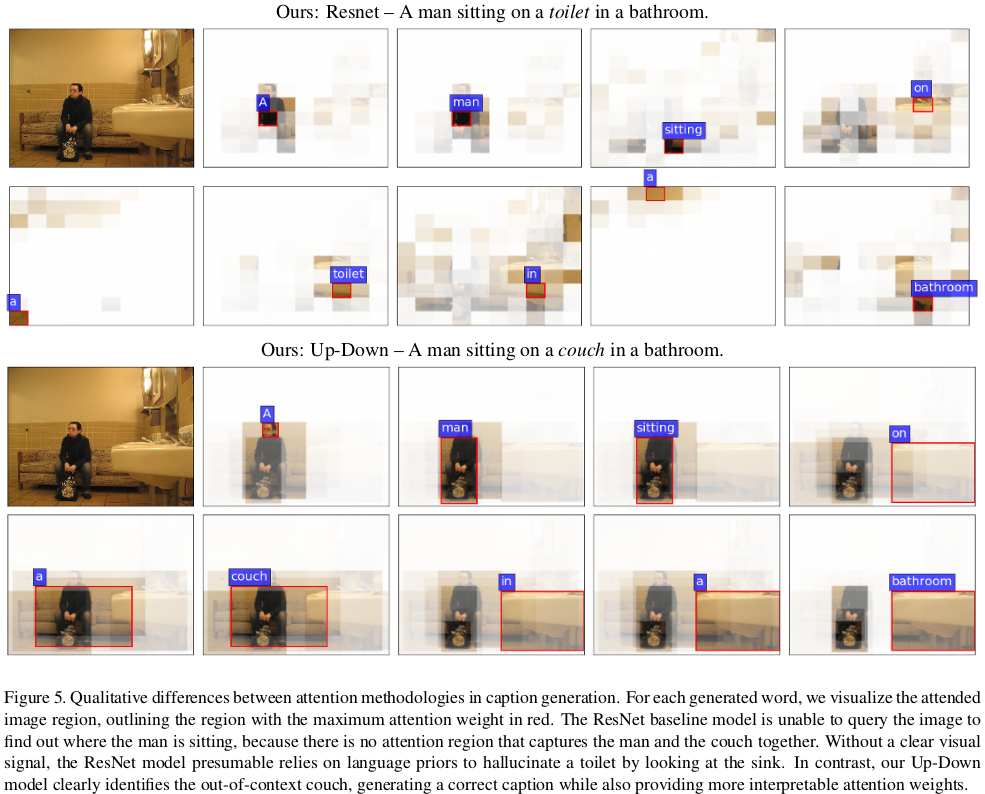（在SCST中也做了out-of-context的图片实验，如果使用attention模型加cross-entropy训练的话是不能准确识别出out-of-context的图片的，加了CIDEr优化以后才能很好地识别出out-of-context的图片。本文这个实验中的Up-Down模型应该也是经过CIDEr优化的，那么这里的结果到底是因为强化学习的效果还是attention的效果呢？）

## 总结